# Bohr’s Atomic Model

Bohr developed a model for hydrogen atom and hydrogen like one–electron species (hydrogenic species). He applied quantum theory in considering the energy of an electron bound to the nucleus.

## Important postulates

 An atom consists of a dense nucleus situated at the centre with the electron revolving around it in circular orbits without emitting any energy. The force of attraction between the nucleus and an electron is equal to the centrifugal force of the moving electron.

 Of the finite number of circular orbits around the nucleus, an electron can revolve only in those orbits whose angular momentum (mvr) is an integral multiple of factor h/2π. As long as an electron is revolving in an orbit it neither loses nor gains energy. Hence these orbits are called stationary states. Each stationary state is associated with a definite amount of energy and it is also known as energy levels. The greater the distance of the energy level from the nucleus, the more is the energy associated with it. The different energy levels are numbered as 1,2,3,4, ( from nucleus onwards) or K,L,M,N etc.

Ordinarily an electron continues to move in a particular stationary state without losing energy. Such a stable state of the atom is called as ground state or normal state.

 If energy is supplied to an electron, it may jump (excite) instantaneously from lower energy (say 1) to higher energy level (say 2,3,4, etc.) by absorbing one or more quanta of energy. This new state of electron is called as excited state. The quantum of energy absorbed is equal to the difference in energies of the two concerned levels. Since the excited state is less stable, atom will lose it’s energy and come back to the ground state.
Energy absorbed or released in an electron jump, (ΔE) is given by,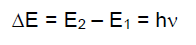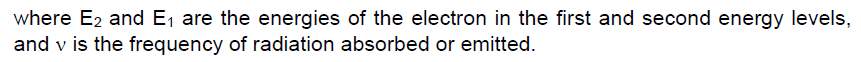[Note: If the energy supplied to hydrogen atom is less than 13.6 eV, it will accept or absorb only those quanta which can take it to a certain higher energy level i.e., all those photons having energy less than or more than a particular energy level will not be absorbed by hydrogen atom. But if energy supplied to hydrogen atom is more than 13.6 eV then all photons are absorbed and excess energy appear as kinetic energy of emitted photo electron].

# Radius and Energy levels of hydrogen atom

Consider an electron of mass ‘m’ and charge ‘e’ revolving around a nucleus of charge Ze (where, Z = atomic number and e is the charge of the proton) with a tangential velocity v. r is the radius of the orbit in which electron is revolving.

By Coulomb’s Law, the electrostatic force of attraction between the moving electron
and nucleus isHence only certain orbits whose radii are given by the above equation are available for the electron. The greater the value of n, i.e., farther the energy level from the nucleus the greater is the radius.# Calculation of energy of an electron

The total energy, E of the electron is the sum of kinetic energy and potential energy.This expression shows that only certain energies are allowed to the electron. Since this energy expression consist of so many fundamental constant, we are giving you the following simplified expressions.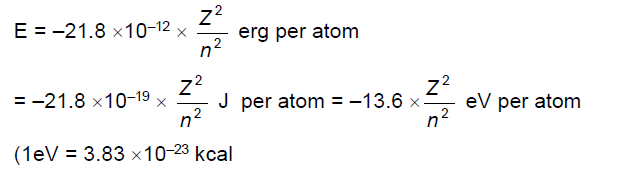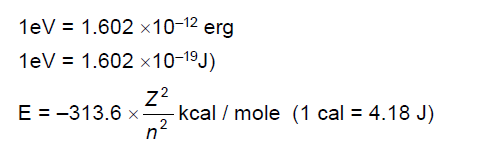The energies are negative since the energy of the electron in the atom is less than the energy of a free electron (i.e., the electron is at infinite distance from the nucleus) which is taken as zero. The lowest energy level of the atom corresponds to n=1, and as the quantum number increases, E becomes less negative.Question: Find out the value of electrostatic potential energy of two electrons separated by 3.0 Å in vacuum. Express your answer in joules and electron volt.

Explanation for hydrogen spectrum by Bohr’s theory
According to the Bohr’s theory electron neither emits nor absorbs energy as long as it stays in a particular orbit. However, when an atom is subjected to electric discharge or high temperature, and electron in the atom may jump from the normal energy level, i.e., ground state to some higher energy level i.e, exited state. Since the life time of the electron in excited state is short, it returns to the ground state in one or more jumps.

During each jump, energy is emitted in the form of a photon of light of definite wavelength or frequency. The frequency of the photon of light thus emitted depends upon the energy difference of the two energy levels concerned (n1, n2) and is given byThe frequencies of the spectral lines calculated with the help of above equation are found to be in good agreement with the experimental values. Thus, Bohr’s theory elegantly explains the line spectrum of hydrogen and hydrogenic species.

Bohr had calculated Rydberg constant from the above equation.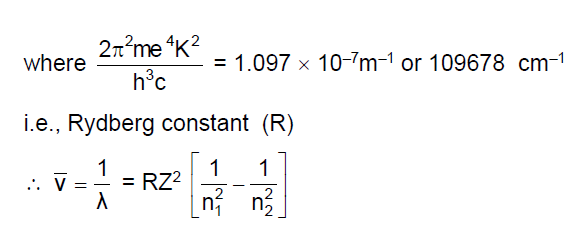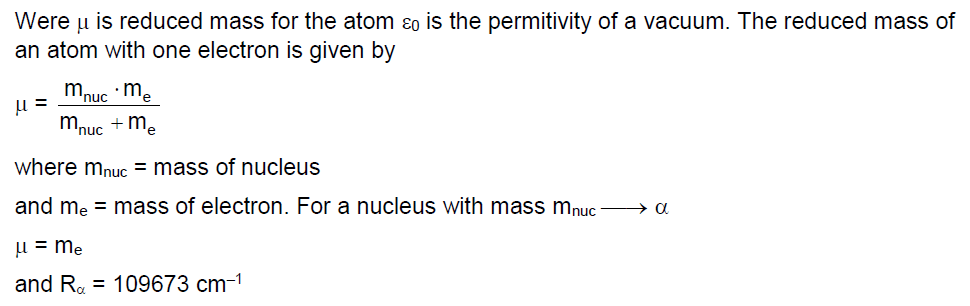CBSE Class 11 Chemistry